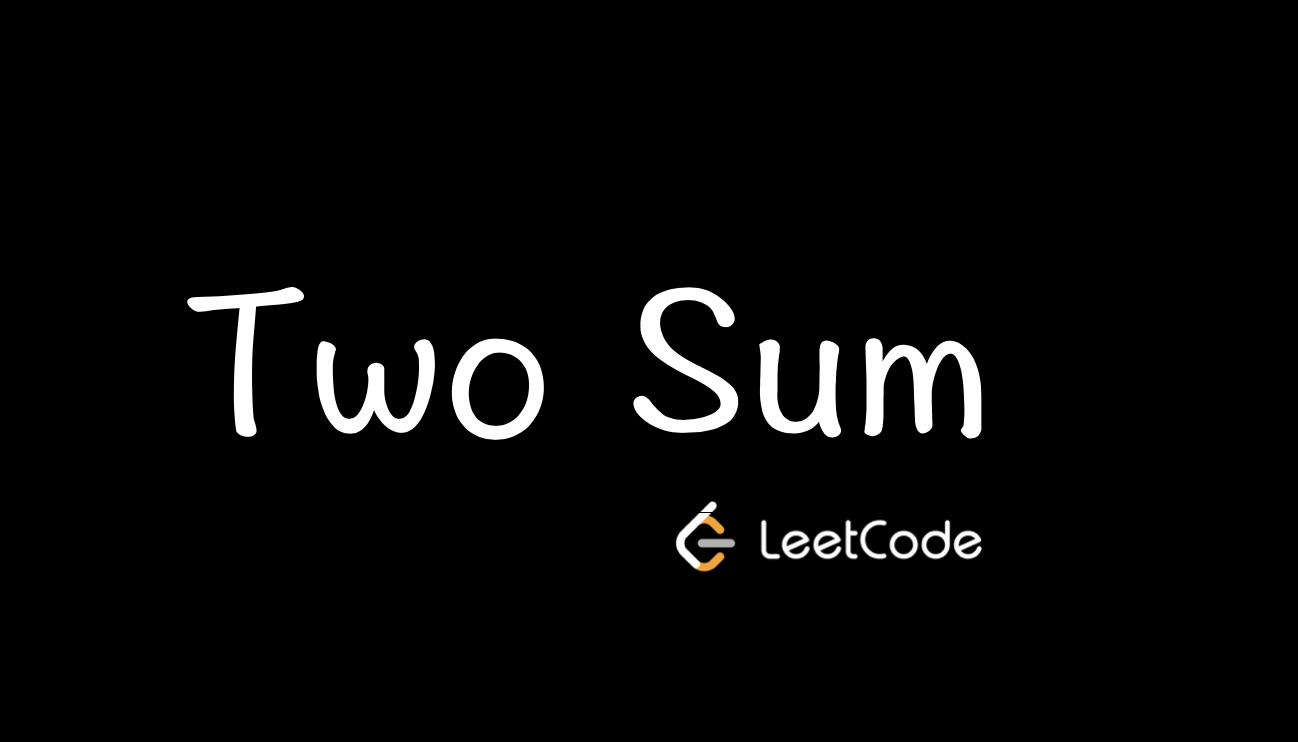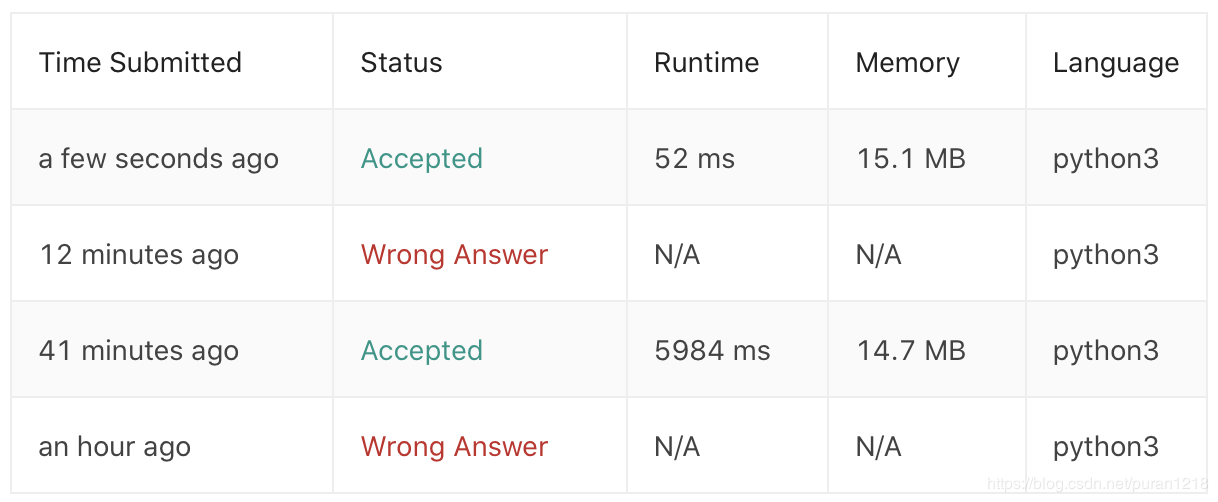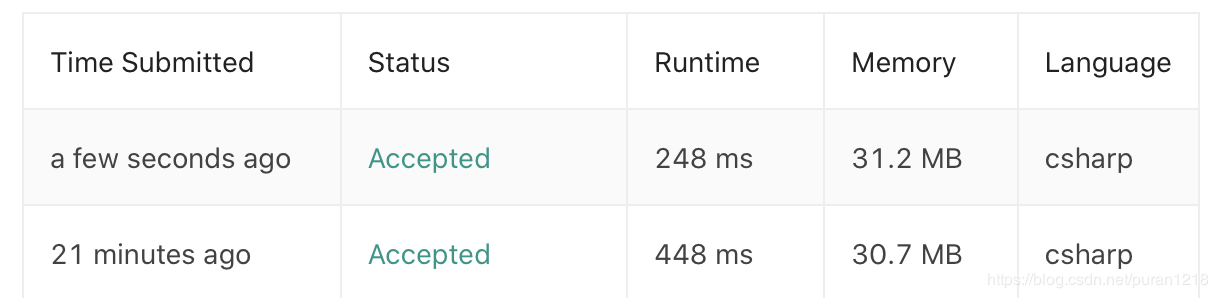# LeetCode | 1. Two Sum 两数之和PuranTwo Sum 是 LeetCode 算法题库中的第一道题，难度为 Easy，题目地址为：https://leetcode.com/problems/two-sum/

## 1. 问题描述

1. 输入的数组只有一个符合条件的解

2. 数组中的元素不能使用两次

Given nums = [2, 7, 11, 15], target = 9,Because nums + nums = 2 + 7 = 9,return [0, 1].

## 2. 解题思路

• 暴力解法：通过两层循环遍历数组以得到所有的组合，将组合数与目标值进行对比，若相同则结束循环（因为假设1的存在）

• 映射：通过增加一个映射表以将循环缩减至一层。遍历数组中的元素，通过目标值与元素的差值与映射表中记录的元素进行对比，若符合则结束循环。

## 3. 知识点

### Python

• 函数注解 Function Annotations

• Map 函数

• Enumerate 函数

#### 函数注解 Function Annotations

def foobar(a: int, b: 'it's b', c: str = 5) -> tuple:return a, b, c
• a: int 这种是注解参数

• c: str = 5 是注解有默认值的参数

• -> tuple 是注解返回值。

#### Map函数

map() 会根据提供的函数对指定序列做映射。

# Return double of n def addition(n):     return n + n   # We double all numbers using map() numbers = (1, 2, 3, 4) result = map(addition, numbers) print(list(result))

#### Enumerate 函数

enumerate()是用来遍历一个可迭代容器(如列表、元组或字符串)中的元素，同时通过一个计数器变量记录当前元素所对应的索引值。

enumerate()语法：enumerate(sequence, [start=0])，其中 start 为下标起始位置。返回枚举对象。

names = ['Bob', 'Alice', 'Guido']for index, value in enumerate(names, 1):    print(f'{index}: {value}')

1: Bob2: Alice3: Guido

### C#

• Dictionary

Dictionary 的一些常用用法：

1. 创建及初始化: var myDictionary = new Dictionary<int, string>();

2. 添加元素: myDictionary.Add(1, "C#");

3. 通过 Key 查找元素: myDictionary.ContainsKey(1))

4. 通过 KeyValuePair 遍历元素：foreach(KeyValuePair<int, string> kvp in myDictionary)

## 4. 代码

### Python实现

class Solution:    def twoSum(self, nums: List[int], target: int) -> List[int]:        length = len(nums)        for i in range(length):            for j in range(i+1, length, 1):                if (nums[i] + nums[j] == target):                    return [i, j]                    break

class Solution:    def twoSum(self, nums: List[int], target: int) -> List[int]:        dict_map = {}        for i, num in enumerate(nums):            diff = target - num            if diff in dict_map:                return [dict_map[diff], i]            dict_map[num] = i### C# 实现

public class Solution {    public int[] TwoSum(int[] nums, int target) {        int[] result = new int;        for (int i = 0; i < nums.GetLength(0); i++)        {            for (int j = i+1; j < nums.GetLength(0); j++)            {                if (nums[i] + nums[j] == target)                {                    result = i;                    result = j;                    break;                }            }        }        return result;    }}

public class Solution {    public int[] TwoSum(int[] nums, int target) {        var dict_map = new Dictionary<int, int>();        for (int i = 0; i < nums.Length; i++)        {            int diff = target - nums[i];            if (dict_map.ContainsKey(diff))            {                return new int[] {dict_map[diff], i};                break;            }            else if (!dict_map.ContainsKey(nums[i]))            {                dict_map.Add(nums[i], i);            }        }        return null;    }}### Puran

GIS从业者，正在往开发的路上小跑。 2018.03.29 加入

## 评论